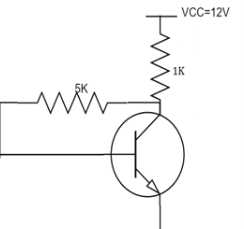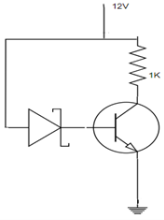Courses

# Test: Collector To Base Bias

## 10 Questions MCQ Test Electronic Devices | Test: Collector To Base Bias

Description
This mock test of Test: Collector To Base Bias for Electrical Engineering (EE) helps you for every Electrical Engineering (EE) entrance exam. This contains 10 Multiple Choice Questions for Electrical Engineering (EE) Test: Collector To Base Bias (mcq) to study with solutions a complete question bank. The solved questions answers in this Test: Collector To Base Bias quiz give you a good mix of easy questions and tough questions. Electrical Engineering (EE) students definitely take this Test: Collector To Base Bias exercise for a better result in the exam. You can find other Test: Collector To Base Bias extra questions, long questions & short questions for Electrical Engineering (EE) on EduRev as well by searching above.
QUESTION: 1

### The collector current (IC) that is obtained in a collector to base biased transistor is_________

Solution:

The collector current is analysed by the DC analysis of a transistor. It involves the DC equivalent circuit of a transistor. The base current is first found and the collector current is obtained from the relation, IC=IBβ.

QUESTION: 2

### The collector to emitter voltage (VCE) is obtained by_________

Solution:

The collector to emitter voltage is obtained in order to find the operating point of a transistor. It is taken when there is no signal applied to the transistor. The point thus obtained lies in the cut off region when the transistor is used as a switch.

QUESTION: 3

### What is the DC characteristic used to prove that the transistor is indeed biased in saturation mode?

Solution:

When in a transistor is driven into saturation, we use VCE(SAT) as another linear parameter. In, addition when a transistor is biased in saturation mode, we have IC < βIB. This characteristic used to prove that the transistor is indeed biased in saturation mode.

QUESTION: 4

The thermal runway is avoided in a collector to base bias because_________

Solution:

The self destruction of a transistor due to increase temperature is called thermal run away. It is avoided by the negative feedback produced by the base resistor in a collector to base bias. The IC which is responsible for the damage is reduced by decreased output signal.

QUESTION: 5

When the temperature is increased, what happens to the collector current after a feedback is given?

Solution:

Before the feedback is applied, when the temperature is increased, the reverse saturation increases. The collector current also increases. When the feedback is applied, the base current increases with decreasing collector current and the thermal runway too.

QUESTION: 6

The demerit of a collector to base bias is_________

Solution:

When the stability factor S=1, the collector resistor value should be very large when compared to the base resistor. So, when RC is large we need to provide large power supply which increases the cost. At the same time, as the base resistor is small we need to provide small power supply.

QUESTION: 7

The negative feedback does good for DC signal by_________

Solution:

The resistor RB can provide negative feedback for both AC and DC signals. The negative feedback for DC signal is done good as it can provide stable operating point. On the other side, the negative feedback is badly done for AC signal by decreasing the voltage gain.

QUESTION: 8

In the circuit, transistor has β =60, VBE=0.7V. Find the collector to emitter voltage drop VCE.Solution:

We know, IC=(VCC-VBE)/RB
By putting the values, we have IC=5.9mA. IE=IC/α. So, IE=5.99mA.
VCE= VCC-RC(IC+IB). We have VCE=6V.

QUESTION: 9

In the circuit shown below, β =100 and VBE=0.7V. The Zener diode has a breakdown voltage of 6V. Find the operating point.Solution:

We know, by KVL -12+(IC+IB)1K+6+VBE=0
We have IE=5.3. IC= αIE=5.24mA. From another loop, -12+IEIK+VBE=0
We have, VCE=12-5.3m*1000=6.7V. Hence the Q point is (6.7V, 5.3mA).

QUESTION: 10

When the β value is large for a given transistor, the IC and VCE values are given by_________

Solution:

The base current IB is zero when β value is large. So, the VCE changes to VCC-RCIC. The collector current IC is changed to (VCC-VBE)/RB from β(VCC-VBE)/(1+ β)RE+ RB.